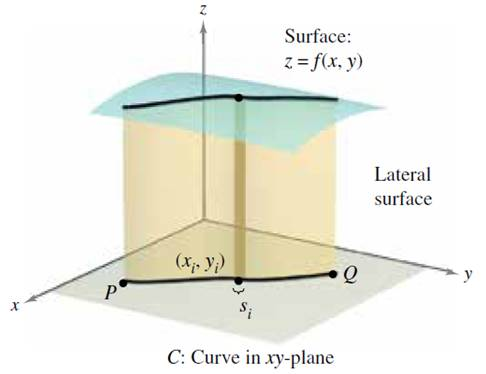Chapter 15.2, Problem 71E### Calculus: Early Transcendental Fun...

7th Edition
Ron Larson + 1 other
ISBN: 9781337552516

#### Solutions

Chapter
Section### Calculus: Early Transcendental Fun...

7th Edition
Ron Larson + 1 other
ISBN: 9781337552516
Textbook Problem

# Lateral Surface Area In Exercises 65-72, find the area of the lateral surface (see figure) over the curve C in the x y-plane and under the surface z = f ( x , y ) , where Lateral surface area = ∫ C f ( x , y ) d s ⋅ .f ( x , y ) = x y , C : y = 1 − x 2 from (1, 0) to (0, 1)

To determine

To Calculate: The area of the lateral surface over the curve C in the xy-plane and under the given surface z=f(x,y)=h where lateral surface area is Cf(x,y)ds. The function is f(x,y)=xy.

Refer to figure in question.

Explanation

Given:

The given surface is f(x,y)=xy and C:y=1x2 from (1,0) to (0,1).

Formula Used:

The integration formula is u2+a2du=12(uu2+a2+a2ln|u+u2+a2|)+C.

And,

u2u2+a2du=18[u(2u2+a2)u2+a2+a4ln|u+u2+a2|]+C

Calculation:

Let x=cost then,

y=1cos2t=sin2t

Usingthis data, the position vector as:

r(t)=xi+yj=costi+sin2tj,0tπ2

On differentiating r(t),

r(t)=sinti+2sintcostjr(t)=sin2t+4sin2tcos2t=sint1+4cos2t

So,

ds=r(t)dt=sint1+4cos2tdt

Thus, lateral surface area,

Cf(x,y)ds=Cxyds=0π2costsin2tsint

### Still sussing out bartleby?

Check out a sample textbook solution.

See a sample solution

#### The Solution to Your Study Problems

Bartleby provides explanations to thousands of textbook problems written by our experts, many with advanced degrees!

Get Started

## Additional Math Solutions

#### Solve the equations in Exercises 126. x4x+1xx1=0

Finite Mathematics and Applied Calculus (MindTap Course List)

#### In Problems 1 and 2, factor out the common monomial factor. 1.

Mathematical Applications for the Management, Life, and Social Sciences

#### If a X b, show that a E(X) b.

Probability and Statistics for Engineering and the Sciences

#### Differentiate the function. B(y) = cy6

Single Variable Calculus: Early Transcendentals

#### Subtract: (4)(+6)

Elementary Technical Mathematics